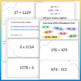# GRADE 4 Treasure Hunt NBT Math - Number & Operations in Base 10 Review ActivitySubject
Resource Type
File Type
Zip (9 MB|12 pages)
Standards
\$3.50
• Product Description
• Standards
***New Resource!***

A fun, engaging activity to review all the Number & Operations in Base Ten (NBT) Common Core State Standards for Grade 4!

Activity Outline:
Students work in pairs or groups to be the first to discover where the treasure is buried.
Each group has a treasure map and has to go around the classroom to find questions stuck in different locations.

When they solve a question they have to cross off the corresponding answer on their treasure hunt map. As there are 16 numbers on the map, once they have been to all 15 questions the remaining number is the location at which the treasure is buried!

- Printable Treasure Map
- 15 CCSS Aligned Questions - to print off and stick around the room
- Complementary PowerPoint Slideshow - Use to set the scene at the beginning and reveal the answer in a fun way at the end!
- Activity instructions

A perfect way to review the NBT topic in an educational but exciting way.

Find whole-number quotients and remainders with up to four-digit dividends and one-digit divisors, using strategies based on place value, the properties of operations, and/or the relationship between multiplication and division. Illustrate and explain the calculation by using equations, rectangular arrays, and/or area models.
Multiply a whole number of up to four digits by a one-digit whole number, and multiply two two-digit numbers, using strategies based on place value and the properties of operations. Illustrate and explain the calculation by using equations, rectangular arrays, and/or area models.
Fluently add and subtract multi-digit whole numbers using the standard algorithm.
Use place value understanding to round multi-digit whole numbers to any place.
Read and write multi-digit whole numbers using base-ten numerals, number names, and expanded form. Compare two multi-digit numbers based on meanings of the digits in each place, using >, =, and < symbols to record the results of comparisons.
Total Pages
12 pages